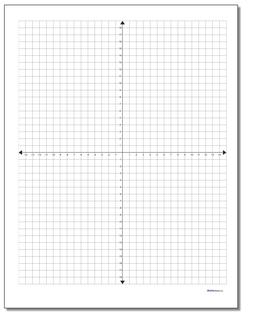# Math Worksheets: Coordinate Plane: Coordinate Plane: Cartesian Standard Graph Paper## Cartesian Standard Graph Paper

PropertyValue
DescriptionCartesian Standard Graph Paper: Printable coordinate planes in inch and metric dimensions in various sizes, great for plotting equations, geometry problems or other similar math problems. These are full four-quadrant graphs. These coordinate planes have x axis and y axis labels along the outer edge of the page.
Resource TypeTemplate